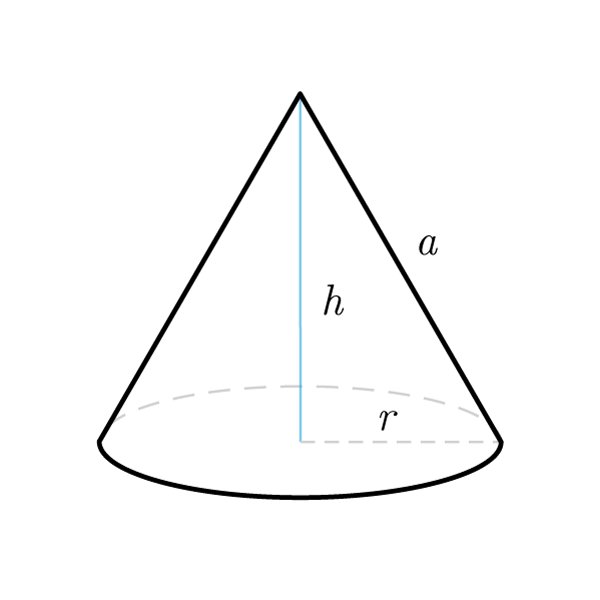# Cone

 Radius $$r$$ Height $$h$$ Apothem $$a$$
$$V = \frac{\pi {r}^{2} h}{3}$$
Volume
$$r = \sqrt{\frac{3 V}{\pi h}}$$
$$h = \frac{3 V}{\pi {r}^{2}}$$
Height
$$V = \frac{A_{base} h}{3}$$
Volume
By using the Pythagoras' Theorem
$$a = \sqrt{{h}^2 + {r}^2}$$
Apothem
$$h = \sqrt{{a}^2 - {r}^2}$$
Height
$$r = \sqrt{{a}^2 - {h}^2}$$
Lateral surface
$$S_{tot} = A_{base} + S_{lat}$$
Total surface
$$S_{lat} = S_{tot} - A_{base}$$
Lateral surface
$$S_{lat} = \pi r a$$
Lateral surface
$$r = \frac{S_{lat}}{\pi a}$$
$$r = \frac{S_{lat}}{\pi r}$$
Apothem
$$A_{base} = \pi {r}^2$$
Base area
$$A_{base} = S_{tot} - S_{lat}$$
Base area
Other formulas
$$2 r = a$$
Equilateral cone

## Definition

A cone is a solid of rotation which is obtained by rotating a right triangle around one of cathetus.

## Properties

1. In a cone - the radius, height and apothem form a right triangle
2. A cone is equivalent (has the same volume) to one third of a cylinder having the same length and radius and height as those of the cone
3. An equilateral cone is a cone in which the diameter and the apothem have the same length## Cone Formulas

Data Formula
Volume V = (π r2 h) / 3
Radius V = √[ (3V) / (π h) ]
Height h = (3V) / (π r2)
Volume V = (Abase × h) / 3
Apothem a = √(h2 + r2)
Height h = √(a2 - r2)
Radius r = √(a2 - h2)
Total surface Stot = Abase + Slat
Lateral surface Slat = Stot - Abase
Lateral surface Slat = π r a
Radius r = Slat / (π a)
Apothem a = Slat / (π r)
Base area Abase = π r2
Base area Abase = Stot - Slat